数据库课程设计-题库管理系统

## 数据库课程设计-题库管理系统*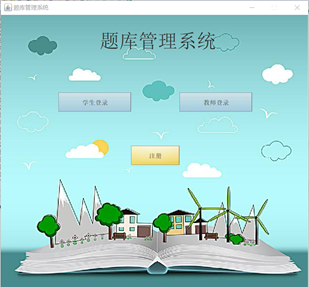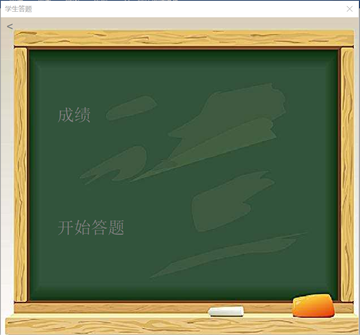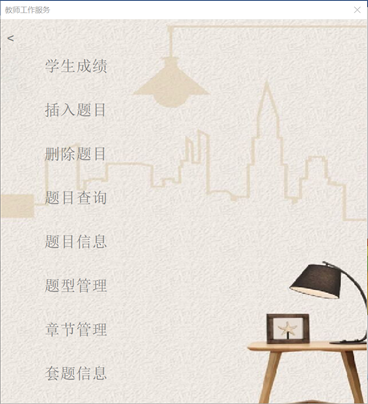1.需求分析. 3
2.数据库设计以及E-R分析. 4
3.结构设计. 4
（1）表结构设计
（2）相关约束
（3）视图
（4）存储过程
（5）触发器
4.JAVA应用程序端. 9
（1）功能设计
（2）界面设计
5.JAVA应用程序端具体实现. 28
6.总结. 35

l  实现题型，题目等基本信息的管理

l  实现章节的管理

l  实现题型的管理

l  能按题型或章节录入课程的习题

l  能够按照题目编号删除题目

l  创建存储过程查询某种题型和各章节的习题数量

l  创建视图查询各题型的数量详细信息

l  创建视图查询各章节的题目数量详细信息

l  创建视图查看套题汇总详细信息

l  习题题号由系统自动生成，从1开始编号

l  可以自动抽题组成套题，习题每抽取一次，要使习题的抽取次数加1（触发器）

l  当题目插入删除时，自动更改题型和各章节题目数量（触发器）

l  实现学生答题系统，并为其录入成绩信息

l  可以查看学生详细信息

l  可以查看已经抽取的套题详细信息

l  建立数据库相关表之间的参照完整性约束。

JAVA应用程序端

（1）

l  学生和老师登录、注册（及其容错）设计

l  教师工作服务界面设计

l 学生答题界面设计

l Java界面细节设计

1初始程序界面：2如果这个学生和老师从来没有进入过这个程序，需要进行注册：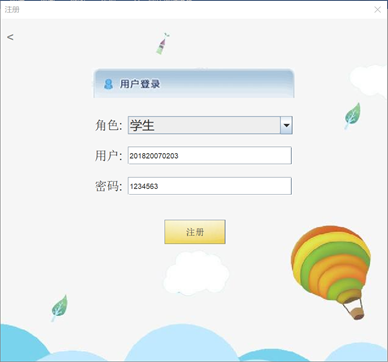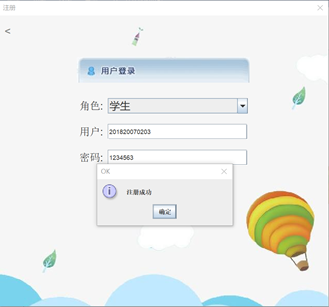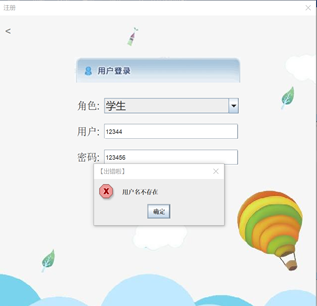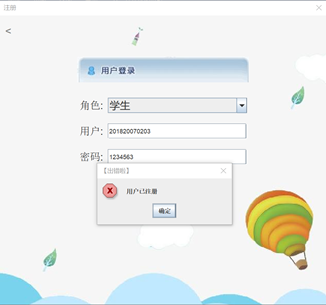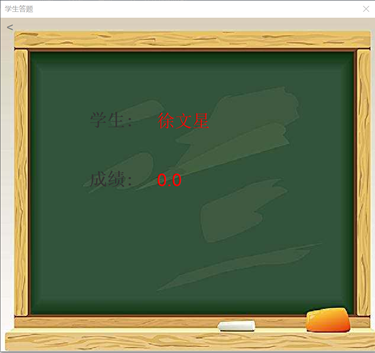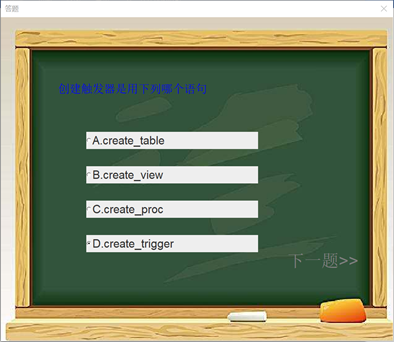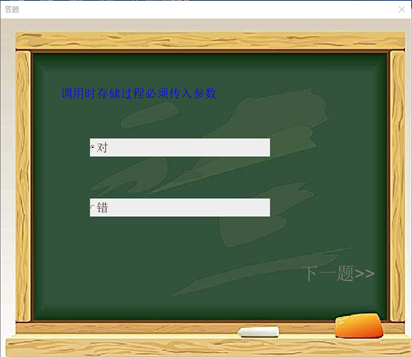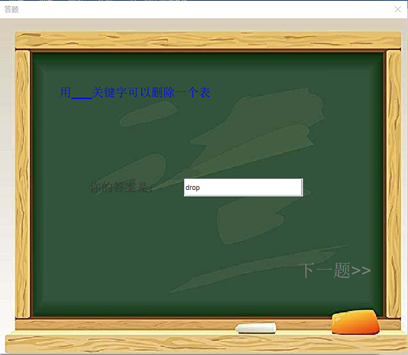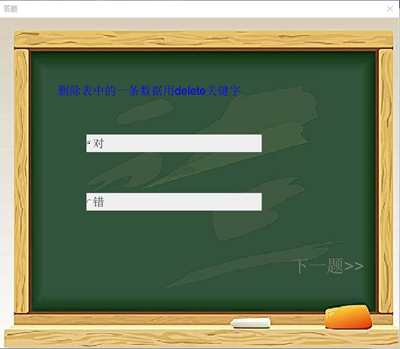![在这里插入图片描述](https://img-blog.csdnimg.cn/20200710203535135.png?x-oss-process=image/watermark,type_ZmFuZ3poZW5naGVpdGk,shadow_10,text_aHR0cHM6Ly9ibG9nLmNzZG4ubmV0L2NhaW5pYW8xXw==,size_16,color_FFFFFF,t_70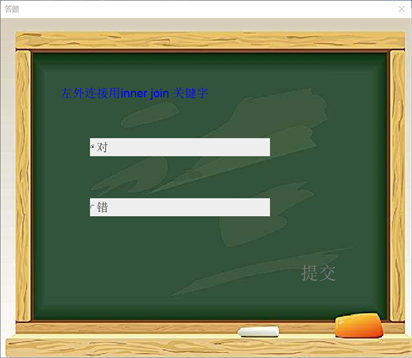教师服务工作界面JAVA应用程序端具体实现

String type = register_JBox_type.getSelectedItem().toString();  //获取下拉列表框中选中的对象的信息

String user = register_field_user.getText();

String passwd = register_field_passwd.getText();

String sql;

if(type.equals("学生")) {

sql ="select * from student";

try {

int flag = 0;  //用于标识判断输入的用户名是否存在

rs = st.executeQuery(sql);

while(rs.next()) {

if(rs.getString("sno").equals(user))

{

flag = 1;

if(rs.getString("passwd") != null) {

}

else {

String   sql2 = "update student set passwd=? where sno=?";

pst = con.prepareStatement(sql2);

pst.setString(2,user);

pst.setString(1,passwd);

pst.executeUpdate();

}

break;

}

}

if(flag == 0) {

}

} catch (SQLException e1) {

e1.printStackTrace();

}

}

if(type.equals("教师")) {

sql ="select * from user_teacher";

try {

int flag = 0;  //用于标识判断输入的用户名是否存在

rs = st.executeQuery(sql);

while(rs.next()) {

if(rs.getString("id").equals(user))

{

flag = 1;

if(rs.getString("passwd") != null) {

}

else {

String
sql2 = "update user_teacher set passwd=? where id=?";
pst = con.prepareStatement(sql2);

pst.setString(2,user);

pst.setString(1,passwd);

pst.executeUpdate();

}

break;

}

}

if(flag == 0) {

}

} catch (SQLException e1) {

e1.printStackTrace();

}

}

String user = field_user.getText();

String passwd = field_passwd.getText();

String sql;

if(button_text.equals("学生登录")) {

sql ="select * from student";

try {

int flag = 0;  //用于标识判断输入的用户名是否存在

rs = st.executeQuery(sql);

while(rs.next()) {

if(rs.getString("sno").equals(user))

{

flag = 1;

if(rs.getString("passwd") == null) {

}

else if(!rs.getString("passwd").equals(passwd)) {

}

else {

new student(field_user.getText()).init();

}

break;

}

}

if(flag == 0) {

}

} catch (SQLException e1) {

e1.printStackTrace();

}

}

if(button_text.equals("教师登录")) {

sql ="select * from user_teacher";

try {

int flag = 0;  //用于标识判断输入的用户名是否存在

rs = st.executeQuery(sql);

while(rs.next()) {

if(rs.getString("id").equals(user))

{

flag = 1;

if(rs.getString("passwd") == null) {

}

else if(!rs.getString("passwd").equals(passwd)) {

}

else

{

new
teacher().init();

}

break;

}

}

if(flag == 0) {

}

} catch (SQLException e1) {

e1.printStackTrace();

}

}

public static String []content;  //题目内容

public static String []type;    //题目类型

public static String []answer_; //因为选择题只需要填A B C D 所以这个为 将选中的单选框的内容的第一个字符作为该选择题的答案

public static float []g;   //该题的分值

if(student.type[n].equals("判断题")||student.type[n].equals("选择题")) {

String a = group.getSelectedCheckbox().getLabel().substring(0,1);

sql = "update student set total_score = total_score + ? where sno = ?";

try {

pst = con.prepareStatement(sql);

pst.setFloat(1,student.g[n]);

pst.setString(2,student_user);

pst.executeUpdate();

}
catch (SQLException e1) {

e1.printStackTrace();

}

}

}

if(student.type[n].equals("计算题")||student.type[n].equals("填空题")) {

String
a = huida.getText();

sql = "update student set total_score = total_score + ?  where sno = ?";
try {

pst = con.prepareStatement(sql);

pst.setFloat(1,student.g[n]);

pst.setString(2,student_user);

pst.executeUpdate();

}
catch (SQLException e1) {

e1.printStackTrace();

}

}

}

if(n<4)
new start_promble(student_user,n+1).init();

05-31
06-15

01-12
01-17
07-26496
12-287万+
03-28295
02-121674
06-27
06-17
03-171万+
05-07cainiao1_

¥2 ¥4 ¥6 ¥10 ¥20余额支付 (余额：-- )扫码支付获取中扫码支付点击重新获取扫码支付1.余额是钱包充值的虚拟货币，按照1:1的比例进行支付金额的抵扣。
2.余额无法直接购买下载，可以购买VIP、C币套餐、付费专栏及课程。余额充值